Next: Brachistochrone Problem (Catenary) Up: b Previous: Brace Notation

## Brachistochrone Problem

Find the curve joining two points along which a particle falling from rest accelerated by gravity travels in the least time. From conservation of Energy,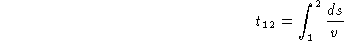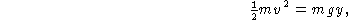so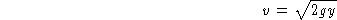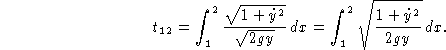The function to be varied is then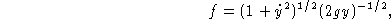and the Euler-Lagrange Differential Equationgives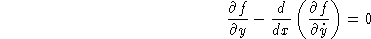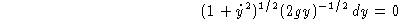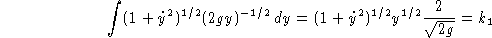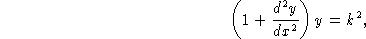where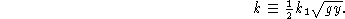Let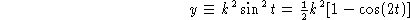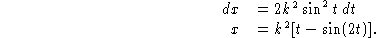Now let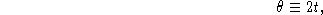then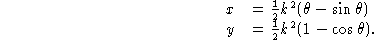If Kinetic Friction is included, terms corresponding to the normal component of weight and the normal component of the acceleration (present because of path curvature) must be included. Including both terms requires a constrained variational technique (Ashby et al. 1975), but including the normal component of weight only gives an elementary solution. The tangent and normal vectors are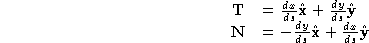Gravity and friction are then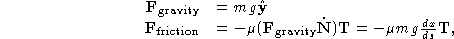and the components along the curve are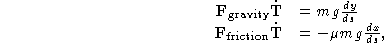so Newton's First Law gives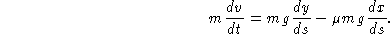But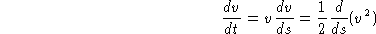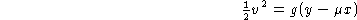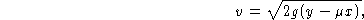so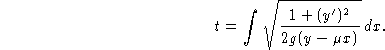Using the Euler-Lagrange Differential Equationgives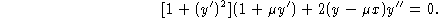This can be reduced to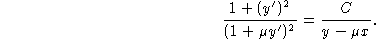Now letting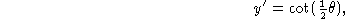the solution is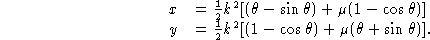References

Ashby, N.; Brittin, W.E.; Love, W.F.; and Wyss, W. ``Brachistochrone with Coulomb Friction.'' Amer. J. Phys. 43, 902-905, 1975.

Haws, L. and Kiser, T. ``Exploring the Brachistochrone Problem.'' Amer. Math. Monthly 102, 328-336, 1995.